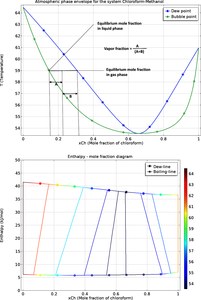# Application Gallery

## Creating Phase Envelopes by Using Equilibrium Calculations

Application ID: 16089

This is a demonstration of how to use the thermodynamics functionality in the COMSOL Multiphysics® software to make flash calculations.

A flash calculation is an equilibrium calculation of a system where several species and phases are present at the same time.

The system in this model is an azeotropic mixture of chloroform and methanol. The plots show the temperature–mole fraction phase envelope and the enthalpy–mole fraction diagram for the system.

Flash calculations are used for processes with vapor–liquid–equilibrium (VLE). A typical process that requires flash calculations is when a feed stream (F) is separated into a vapor (V ) and liquid (L) product. In principle, flash calculations are straightforward and involve combining the VLE equations with the component mass balances, and in some cases the energy balance.

Some flash calculations are (with a comment on their typical numerical solution or usage):

1. Bubble point at given T
2. Bubble point at given p
3. Dew point at given T
4. Dew point at given p (need to iterate on T)
5. Flash at given p and T (relatively easy)
6. Flash at given p and H ("standard" flash, e.g., for a flash tank after a valve)
7. Flash at given p and S (e.g., for condensing turbine)
8. Flash at given U and V (e.g., for dynamic simulation of an adiabatic flash drum)This model example illustrates applications of this type that would nominally be built using the following products: